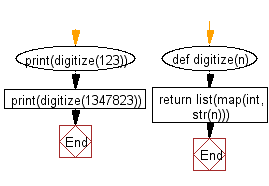﻿ Python: Convert a given number (integer) to a list of digits - w3resource# Python: Convert a given number (integer) to a list of digits

## Python List: Exercise - 234 with Solution

Write a Python program to convert a given number (integer) to a list of digits.

Use map() combined with int on the string representation of n and return a list from the result.

Sample Solution:

Python Code:

``````def digitize(n):
return list(map(int, str(n)))
print(digitize(123))
print(digitize(1347823))
```
```

Sample Output:

```[1, 2, 3]
[1, 3, 4, 7, 8, 2, 3]
```

Flowchart:## Visualize Python code execution:

The following tool visualize what the computer is doing step-by-step as it executes the said program:

Python Code Editor:

Have another way to solve this solution? Contribute your code (and comments) through Disqus.

What is the difficulty level of this exercise?

Test your Python skills with w3resource's quiz

﻿

## Python: Tips of the Day

Floor Division:

When we speak of division we normally mean (/) float division operator, this will give a precise result in float format with decimals.

For a rounded integer result there is (//) floor division operator in Python. Floor division will only give integer results that are round numbers.

```print(1000 // 300)
print(1000 / 300)```

Output:

```3
3.3333333333333335```